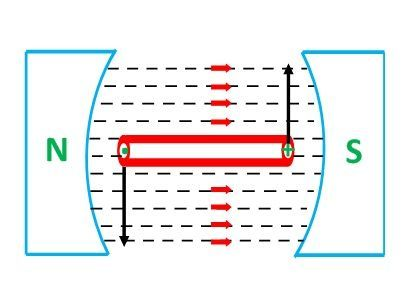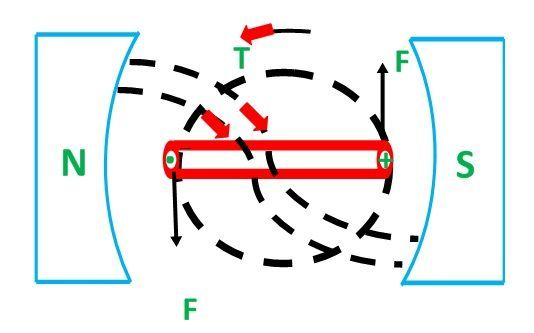# Synchronous Machine

Synchronous Machine constitutes of both synchronous motors as well as synchronous generators. An AC system has some advantages over a DC system. Therefore, the AC system is exclusively used for the generation, transmission, and distribution of electric power. The machine which converts mechanical power into AC electrical power is called a Synchronous Generator or Alternator. However, if the same machine can be operated as a motor is known as Synchronous Motor.

Synchronous machine is an AC machine whose satisfactory operation depends upon the maintenance of the following relationship.Where,

• Ns is the synchronous speed in revolution per minute (r.p.m)
• f is the supply frequency
• P is the number of poles of the machine.

When connected to an electric power system, a synchronous machine always maintains the above relationship shown in equation (1).

If the synchronous machine working as a motor fails to maintain the average speed (Ns) the machine will not develop sufficient torque to maintain its rotation and will stop. Then the motor is said to be Pulled Out of Step.

In case, when the synchronous machine is operating as a generator, it has to run at a fixed speed called Synchronous speed to generate the power at a particular frequency. As all the appliances or machines are designed to operate at this frequency. In some countries, the value of the frequency is 50 hertz.

## Basic Principles of Synchronous Machine

A synchronous machine is just an electromechanical transducer that converts mechanical energy into electrical energy or vice versa. The fundamental phenomenon or law which makes these conversions possible is known as the Law of Electromagnetic Induction and Law of interaction.

The detailed description is explained below.

### Law of Electro-Magnetic Induction

This law is also called Faraday’s First Law of Electromagnetic Induction. This law relates to the production of emf, i.e.; emf is induced in a conductor whenever it cuts across the magnetic field as shown below:

###Law of Interaction

This law relates to the production of force or torque, i.e., whenever a current-carrying conductor is placed in the magnetic field, by the interaction of the magnetic field produced by the current-carrying conductor and the main field, force is exerted on the conductor producing torque. The figure is shown below:### Three-Phase Synchronous Machine

• The machine which is used in the household appliance such as the small machine used in air coolers, refrigeration, fans, air conditioners, etc.
• However, large AC machines are three-phase type synchronous machines because of the following reasons.
• For the same size of the frame, three-phase machines have nearly 1.5 times the output than that of the single-phase machine.
• Three-phase power is transmitted and distributed more economical than single-phase power.
• Three-phase motors are self-starting (except synchronous motors).
• Three-phase motors have an absolute uniform continuous torque, whereas, single-phase motors have pulsating torque.

In a small synchronous machine, the fielding winding is placed on the stator, and the armature winding is placed on the rotor whereas for the large synchronous machine the field winding is placed on the rotor, and the armature winding is placed on the stator.